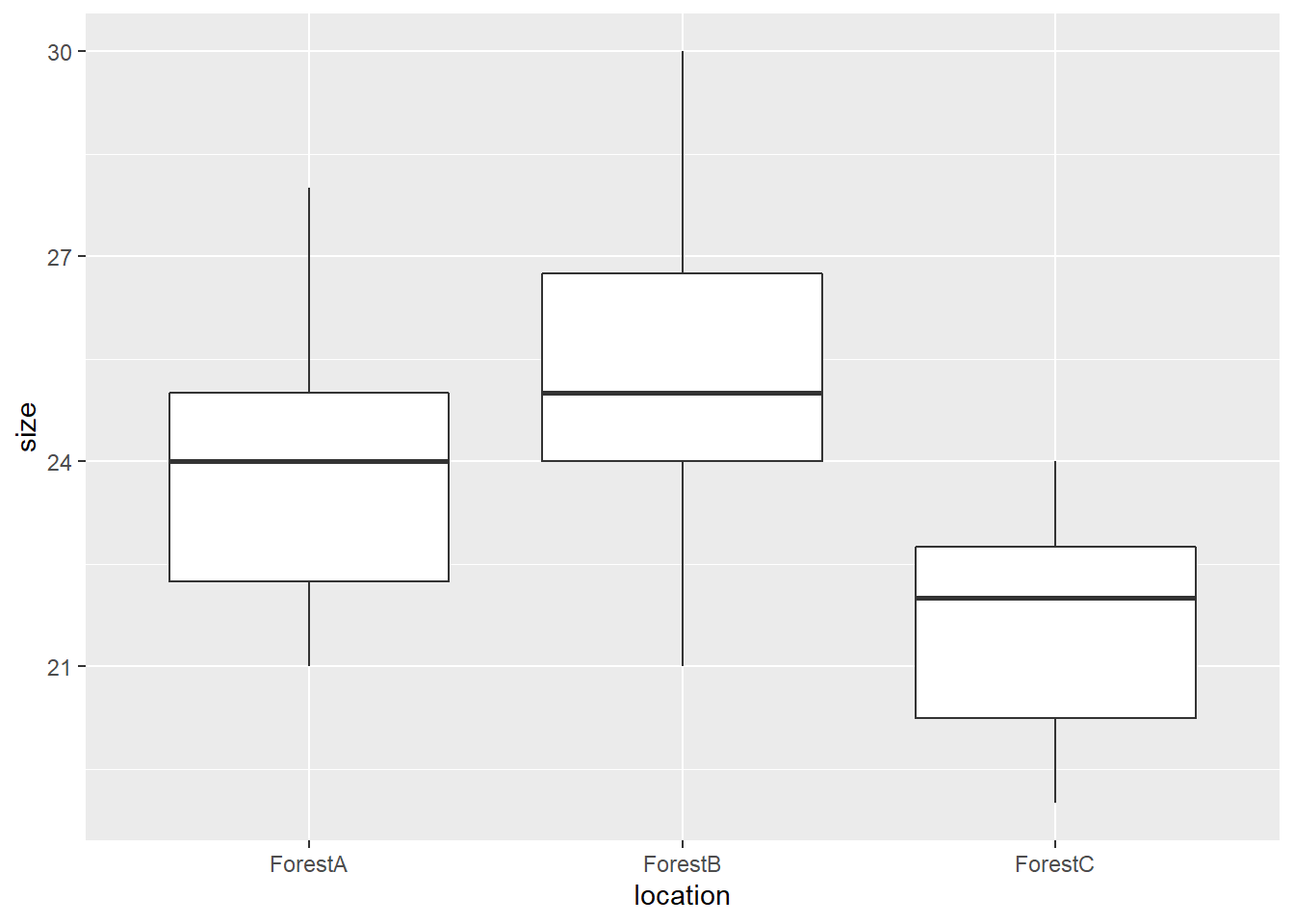One-way ANOVA is a parametric test designed to compare the means of two or more samples. The null hypothesis H0 states that the means of all samples to be tested are equal. The test returns an F-statistic and a p-value which will help you decide whether or not to reject the null hypothesis.

One of the options to perform a one-way ANOVA in R is to run the function lm(); followed by anova(). This option fits a linear model and will work in virtually all cases.

Let’s take an example. Here, let’s say that we want to check whether the average size of blue ground beetles (Carabus intricatus) differs depending on their location. We consider 3 different locations, for example 3 forests beautifully named A, B and C. In each location, we measure the size (in millimeters) of 10 individuals.

To create the corresponding dataframe in R, use the following code:

# response variable
size <- c(25,22,28,24,26,24,22,21,23,25,26,30,25,24,21,27,28,23,25,24,20,22,24,23,22,24,20,19,21,22)

# predictor variable
location <- as.factor(c(rep("ForestA",10), rep("ForestB",10), rep("ForestC",10)))

# dataframe
my.dataframe <- data.frame(size,location)

It is always nice and useful to start by visualizing the whole dataset, so let’s plot the data:### Assumptions

The assumptions are:

• independence of observations (each individual is represented by 1 entry/measurement ONLY)
• normality of distribution (to be tested for each group, for example with the Shapiro-Wilk test)
• homogeneity of variance (to be tested with, for example, Levene’s test)
• groups contain no outliers.

### Running the test

The syntax is lm(response ~ predictor, data = dataframe) where response is the response variable, predictor is the predictor variable or factor (which categorizes the observations) and dataframe the name of the dataframe that contains the data. We first need to fit the linear model with lm() and then we store it in the object model. We then compute and display the table for the analysis using anova():

model <- lm(size ~ location, data = my.dataframe)
anova(model)
## Analysis of Variance Table
##
## Response: size
##           Df  Sum Sq Mean Sq F value   Pr(>F)
## location   2  66.467  33.233  7.1101 0.003307 **
## Residuals 27 126.200   4.674
## ---
## Signif. codes:  0 '***' 0.001 '**' 0.01 '*' 0.05 '.' 0.1 ' ' 1

This output provides you with the F-value (7.1101) and the corresponding p-value (0.003307). The hypothesis H0 stating that the means of the groups are equal is thus to be rejected.

But this does not tell us anything about the groups which means are significantly different…

Indeed, the ANOVA needs to be followed by another test if we want to check which of the groups are different from the others. For that, we will need a post-hoc test, and these three options will help you do that:

### Alternative

The non-parametric Kruskal-Wallis test is a good alternative to the one-way ANOVA when the assumption of normality of distribution is violated.